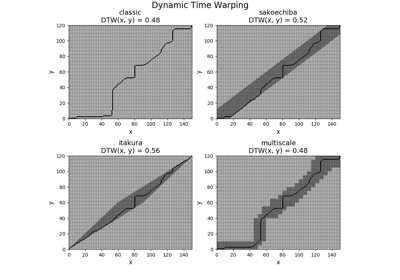# `pyts.metrics`.dtw¶

`pyts.metrics.``dtw`(x=None, y=None, dist='square', method='classic', options=None, precomputed_cost=None, return_cost=False, return_accumulated=False, return_path=False)[source]

Dynamic Time Warping (DTW) distance between two samples.

Parameters: Returns: x : array-like, shape = (n_timestamps_1,) First array. Ignored if `dist == 'precomputed'`. y : array-like, shape = (n_timestamps_2,) Second array. Ignored if `dist == 'precomputed'`. dist : ‘square’, ‘absolute’, ‘precomputed’ or callable (default = ‘square’) Distance used. If ‘square’, the squared difference is used. If ‘absolute’, the absolute difference is used. If callable, it must be a function with a numba.njit() decorator that takes as input two numbers (two arguments) and returns a number. If ‘precomputed’, `precomputed_cost` must be the cost matrix and `method` must be ‘classic’, ‘sakoechiba’ or ‘itakura’. method : str (default = ‘classic’) Method used. Should be one of ‘classic’: Classic DTW ‘sakoechiba’: DTW with Sakoe-Chiba band constraint ‘itakura’: DTW with Itakura parallelogram constraint ‘multiscale’: MultiscaleDTW ‘fast’: FastDTW options : None or dict (default = None) Dictionary of method options ‘classic’: None ‘sakoechiba’: window_size (int or float) ‘itakura’: max_slope (float) ‘multiscale’: resolution (int) and radius (int) ‘fast’: radius (int) For more information on these parameters, see the Other Parameters section. precomputed_cost : array-like, shape = (n_timestamps_1, n_timestamps_2) (default = None). Precomputed cost matrix between the time series. Ignored if `dist != 'precomputed'`. return_cost : bool (default = False) If True, the cost matrix is returned. return_accumulated : bool (default = False) If True, the accumulated cost matrix is returned. return_path : bool (default = False) If True, the optimal path is returned. dist : float The DTW distance between the two arrays. cost_mat : ndarray, shape = (n_timestamps_1, n_timestamps_2) Cost matrix. Only returned if `return_cost=True`. acc_cost_mat : ndarray, shape = (n_timestamps_1, n_timestamps_2) Accumulated cost matrix. Only returned if `return_accumulated=True`. path : ndarray, shape = (2, path_length) The optimal path along the cost matrix. The first row consists of the indices of the optimal path for x while the second row consists of the indices of the optimal path for y. Only returned if `return_path=True`. window_size : float or int (default = 0.1) The window size above and below the diagonale. If float, window_size must be between 0 and 1, and the actual window size will be computed as ``ceil(window_size * max((n_timestamps_1, n_timestamps_2) - 1))`. If int, window_size must be the largest temporal shift allowed. Each cell whose distance with the diagonale is lower than or equal to ‘window_size’ becomes a valid cell for the path. max_slope : float (default = 2.) Maximum slope for the parallelogram. resolution : int (default = 2) The resolution level. radius : int (default = 0) The radius used to expand the constraint region. The optimal path computed at the resolution level is expanded with radius cells to the top, bottom, left and right of every cell belonging to the optimal path. It is computed at the resolution level.

References

  H. Sakoe and S. Chiba, “Dynamic programming algorithm optimization for spoken word recognition”. IEEE Transactions on Acoustics, Speech, and Signal Processing, 26(1), 43-49 (1978).
  F. Itakura, “Minimum prediction residual principle applied to speech recognition”. IEEE Transactions on Acoustics, Speech, and Signal Processing, 23(1), 67–72 (1975).
  M. Müller, H. Mattes and F. Kurth, “An efficient multiscale approach to audio synchronization”. International Conference on Music Information Retrieval, 6(1), 192-197 (2006).
  S. Salvador ans P. Chan, “FastDTW: Toward Accurate Dynamic Time Warping in Linear Time and Space”. KDD Workshop on Mining Temporal and Sequential Data, 70–80 (2004).

Examples

```>>> from pyts.metrics import dtw
>>> x = [0, 1, 1]
>>> y = [2, 0, 1]
>>> dtw(x, y, method='sakoechiba', options={'window_size': 0.5})
2.0
```

## Examples using `pyts.metrics.dtw`¶Dynamic Time Warping